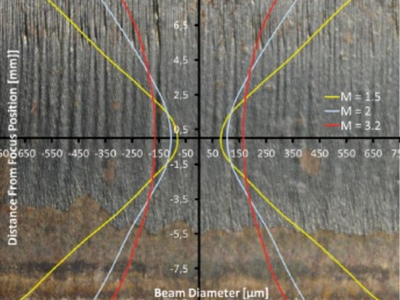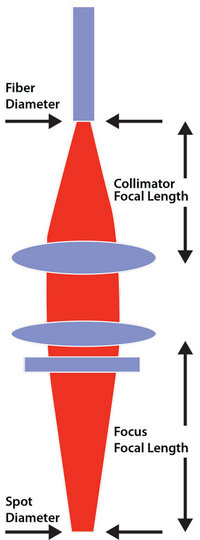# How to Calculate Magnification of Cutting Head?

Time: Oct 11,2017 Author: Sintec Optronics View: 424The laser cutting head magnification M can be calculated from:

M = f(focal unit) / f(collimaton unit) = d(focal diameter) / d(core diameter of fiber)

Focal diameter can be estimated based on above formula:

d(focal diameter) = M * d(core diameter of fiber)

For example, cutting head magnification M=1.5, fiber diameter = 100µm;

d(focal diameter) = 1.5 * 100 = 150µmSource of pictures:

Laser Technik Journal

www.thefabricator.com